Mean

Chapter 14 Class 10 Statistics
Concept wise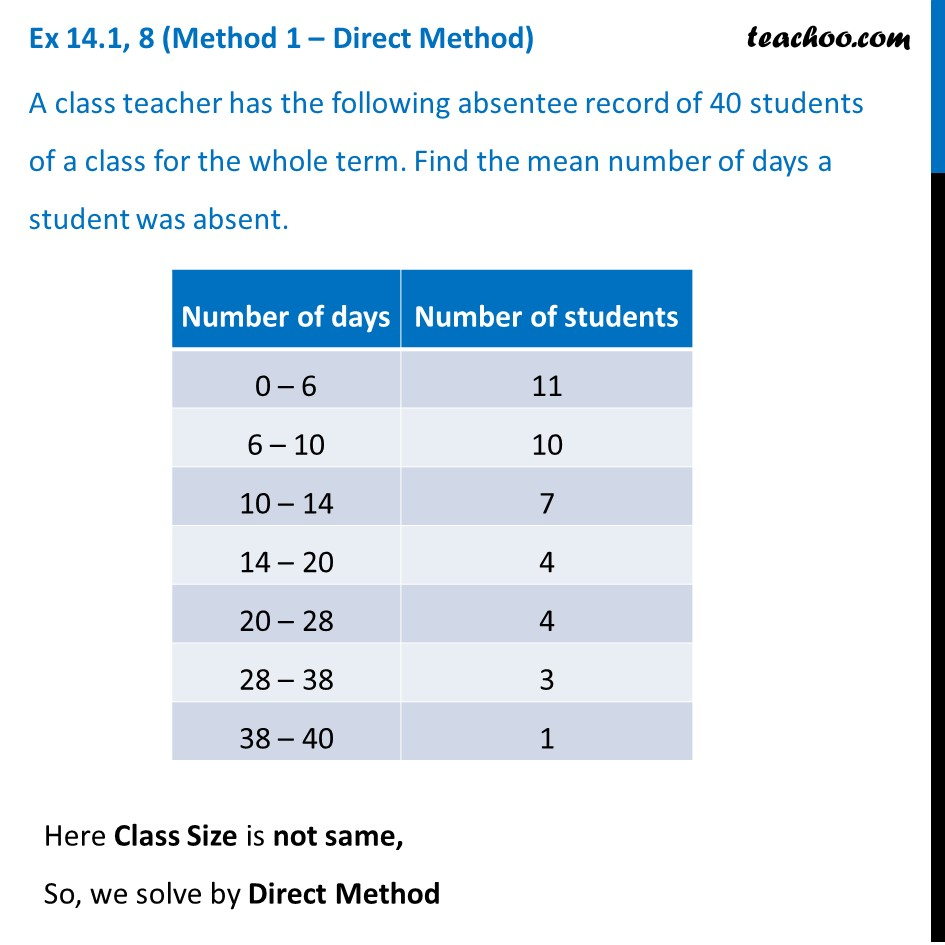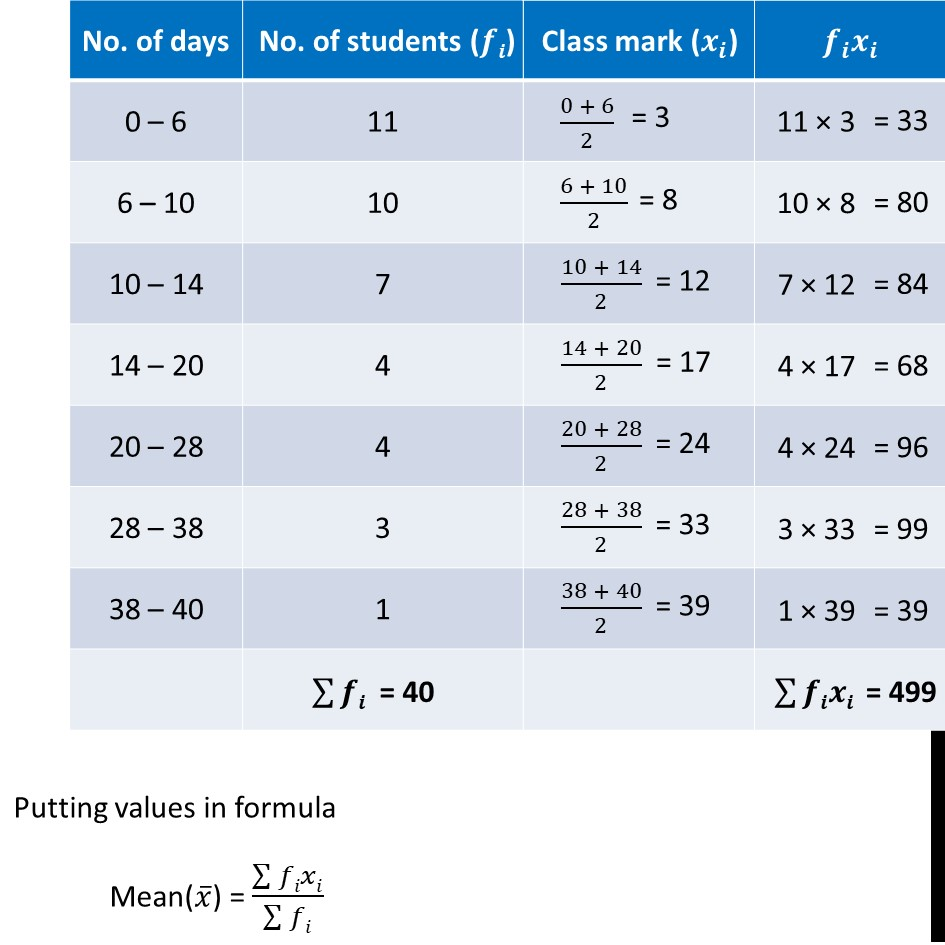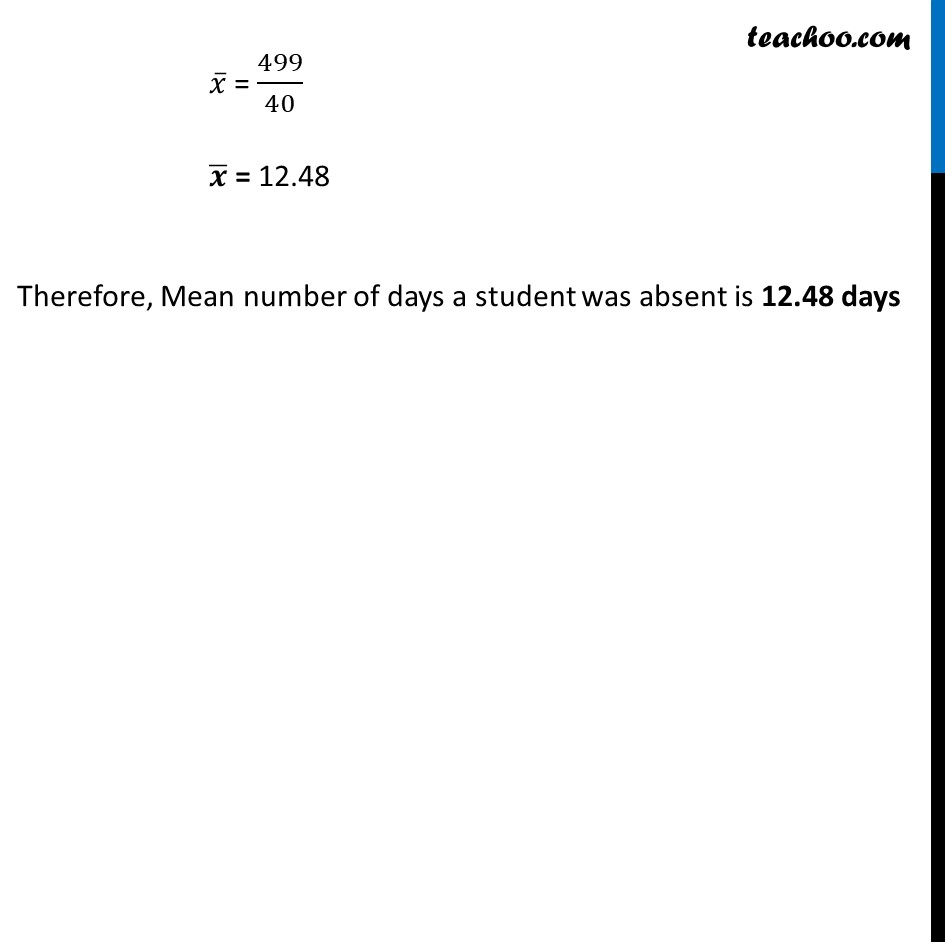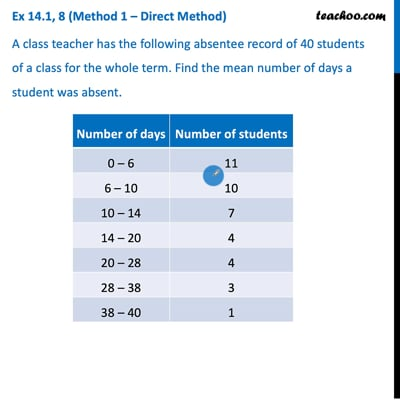This video is only available for Teachoo black users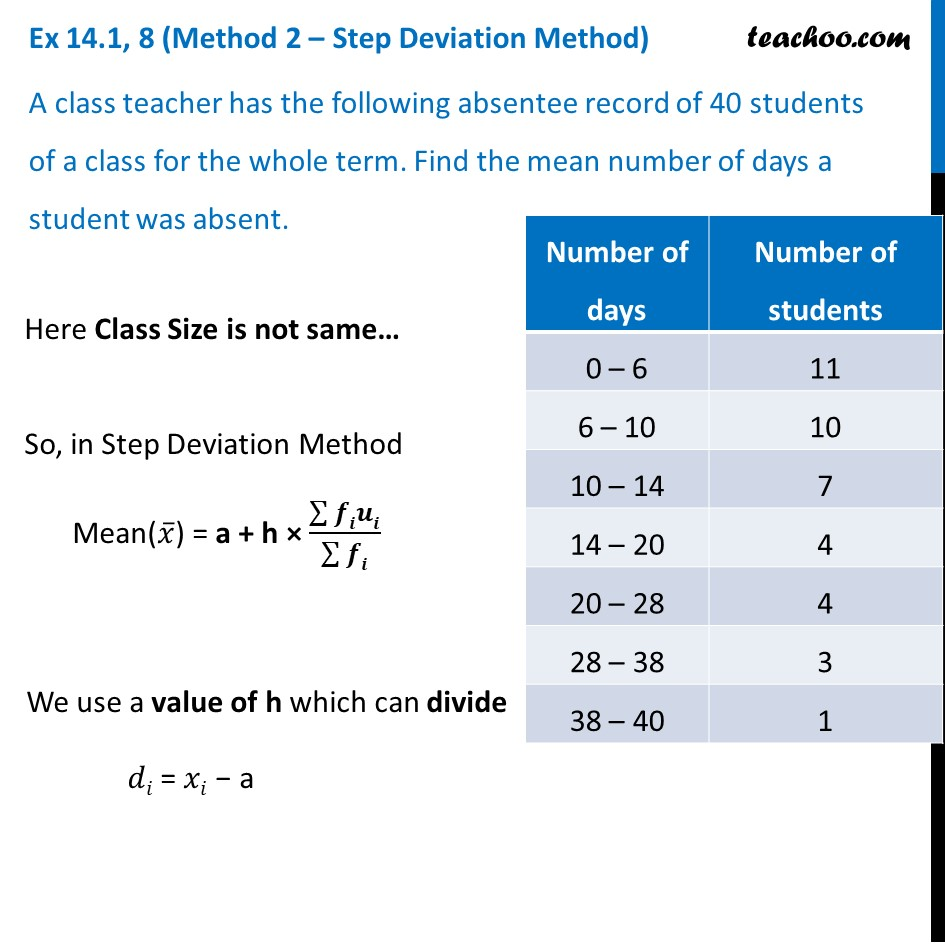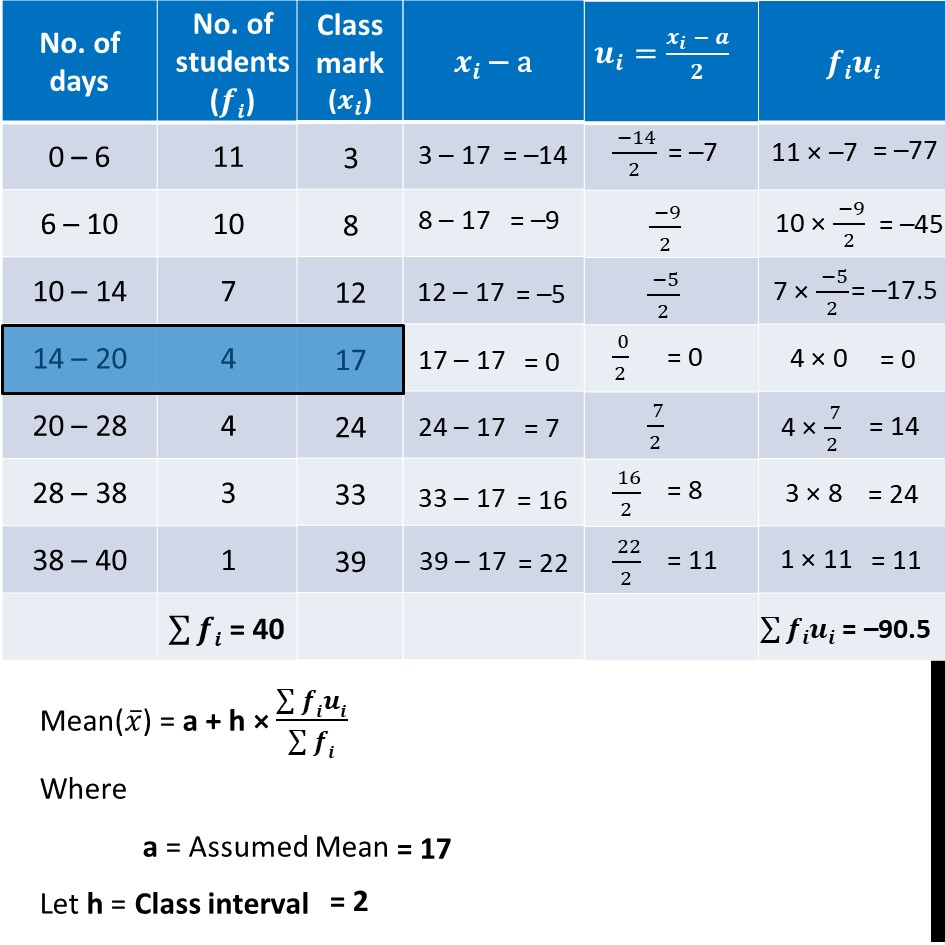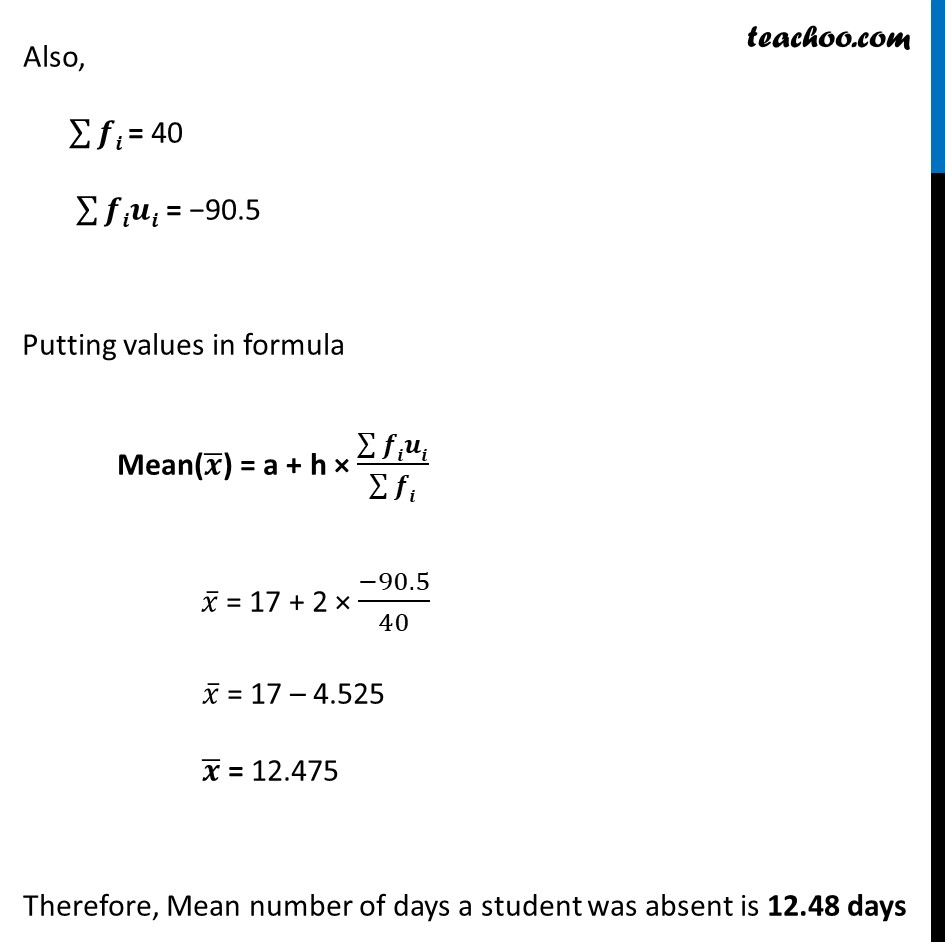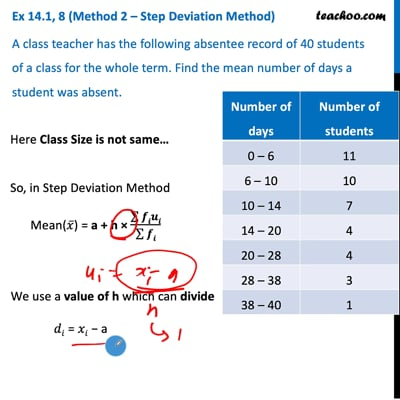This video is only available for Teachoo black users

Introducing your new favourite teacher - Teachoo Black, at only ₹83 per month

### Transcript

Ex 14.1, 8 (Method 1 – Direct Method) A class teacher has the following absentee record of 40 students of a class for the whole term. Find the mean number of days a student was absent. Here Class Size is not same, So, we solve by Direct Method Putting values in formula Mean(𝑥 ̅) = (∑▒𝑓𝑖𝑥𝑖)/(∑▒𝑓𝑖) 𝑥 ̅ = 499/40 𝒙 ̅ = 12.48 Therefore, Mean number of days a student was absent is 12.48 daysEx 14.1, 8 (Method 2 – Step Deviation Method) A class teacher has the following absentee record of 40 students of a class for the whole term. Find the mean number of days a student was absent. Here Class Size is not same… So, in Step Deviation Method Mean(𝑥 ̅) = a + h × (∑▒𝒇𝒊𝒖𝒊)/(∑▒𝒇𝒊) We use a value of h which can divide 𝑑𝑖 = 𝑥𝑖 − a Mean(𝑥 ̅) = a + h × (∑▒𝒇𝒊𝒖𝒊)/(∑▒𝒇𝒊) Where a = Assumed Mean Let h = Class interval Also, ∑▒𝒇𝒊 = 40 ∑▒𝒇𝒊𝒖𝒊 = −90.5 Putting values in formula Mean(𝒙 ̅) = a + h × (∑▒𝒇𝒊𝒖𝒊)/(∑▒𝒇𝒊) 𝑥 ̅ = 17 + 2 × (−90.5)/40 𝑥 ̅ = 17 – 4.525 𝒙 ̅ = 12.475 Therefore, Mean number of days a student was absent is 12.48 days